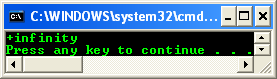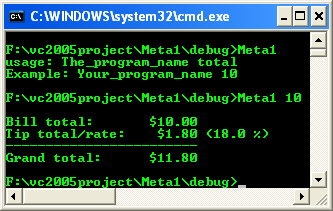# The .NET Framework Class Library 5

The topics in this part are listed below. The code snippets if any are based on the new C++ .NET syntaxes. The code snippets if any are based on the new C++ .NET syntaxes.

2. # Console::WriteLine Examples

 Floating-Point Types   The Single and Double types implement IEEE-754 floating-point arithmetic. For the uninitiated, IEEE-754 floating-point arithmetic means that every operation has a defined result, so you never get a divide-by-zero error when doing floating-point math; instead, you get an answer of infinity. The floating-point classes have values to represent positive and negative infinity and “not a number”, as well as methods to test for them, as shown in the following example: #include "stdafx.h"   using namespace System;   int main(array ^args) {       double top = 10.0;       double bottom = 0.0;         double result = top/bottom;         if (result == Double::PositiveInfinity)             Console::WriteLine("+infinity");       else if (result == Double::NegativeInfinity)             Console::WriteLine("-infinity");       else if (result == Double::NaN)             Console::WriteLine("NaN");         // The Visual C++ compiler supports comparisons of not a number (NAN)       // items in an IEEE-compliant manner. If x is NAN and y is not NAN:       // (x != x) == true       // (x == x) == false       // (y > x) == false       // (y < x) == false       // NAN ordering tests always return false:       // NAN [<, <=, >, >=] [any_number] will be false.       return 0; }   Output:Figure 13

Console::WriteLine Examples

The Console::WriteLine method of the .NET Framework Class Library writes the specified data, followed by the current line terminator, to the standard output stream. The overloaded list of the Console::WriteLine() is given in the following table.

 Name Description Console::WriteLine() Writes the current line terminator to the standard output stream. Supported by the .NET Compact Framework. Console::WriteLine(Boolean) Writes the text representation of the specified Boolean value, followed by the current line terminator, to the standard output stream. Console::WriteLine(Char) Writes the specified Unicode character, followed by the current line terminator, value to the standard output stream. Supported by the .NET Compact Framework. Console::WriteLine(Char[]) Writes the specified array of Unicode characters, followed by the current line terminator, to the standard output stream. Supported by the .NET Compact Framework. Console::WriteLine(Decimal) Writes the text representation of the specified Decimal value, followed by the current line terminator, to the standard output stream. Console::WriteLine(Double) Writes the text representation of the specified double-precision floating-point value, followed by the current line terminator, to the standard output stream. Console::WriteLine(Int32) Writes the text representation of the specified 32-bit signed integer value, followed by the current line terminator, to the standard output stream. Supported by the .NET Compact Framework. Console::WriteLine(Int64) Writes the text representation of the specified 64-bit signed integer value, followed by the current line terminator, to the standard output stream. Console::WriteLine(Object) Writes the text representation of the specified object, followed by the current line terminator, to the standard output stream. Supported by the .NET Compact Framework. Console::WriteLine(Single) Writes the text representation of the specified single-precision floating-point value, followed by the current line terminator, to the standard output stream. Console::WriteLine(String) Writes the specified string value, followed by the current line terminator, to the standard output stream. Supported by the .NET Compact Framework. Console::WriteLine(UInt32) Writes the text representation of the specified 32-bit unsigned integer value, followed by the current line terminator, to the standard output stream. Console::WriteLine(UInt64) Writes the text representation of the specified 64-bit unsigned integer value, followed by the current line terminator, to the standard output stream. Console::WriteLine(String, Object) Writes the text representation of the specified object, followed by the current line terminator, to the standard output stream using the specified format information. Supported by the .NET Compact Framework. Console::WriteLine(String, Object[]) Writes the text representation of the specified array of objects, followed by the current line terminator, to the standard output stream using the specified format information. Supported by the .NET Compact Framework. Console::WriteLine(Char[], Int32, Int32) Writes the specified subarray of Unicode characters, followed by the current line terminator, to the standard output stream. Console::WriteLine (String, Object, Object) Writes the text representation of the specified objects, followed by the current line terminator, to the standard output stream using the specified format information. Supported by the .NET Compact Framework. Console::WriteLine (String, Object, Object, Object) Writes the text representation of the specified objects, followed by the current line terminator, to the standard output stream using the specified format information. Supported by the .NET Compact Framework. Console::WriteLine (String, Object, Object, Object, Object) Writes the text representation of the specified objects and variable length parameter list, followed by the current line terminator, to the standard output stream using the specified format information. Table 7

Let try the program examples. Create a new CLR Console Application, give your desired project name and try the following code sample.

#include "stdafx.h"

// This program run at command prompt

// For System::Console::WriteLine

using namespace System;

int main()

{

// Get the command line arguments

array<String^>^args = Environment::GetCommandLineArgs();

const double tipRate = 0.18;

double billTotal;

// Check the command line argument input

if (args->Length != 2)

{

Console::WriteLine("usage: The_program_name total");

Console::WriteLine("Example: Your_program_name 10");

return 1;

}

else

{

try

{

billTotal = Double::Parse(args);

}

catch (FormatException^)

{

Console::WriteLine("usage: Your_program_name total" );

Console::WriteLine("Example: Your_program_name 10" );

return 1;

}

double tip = billTotal * tipRate;

Console::WriteLine();

Console::WriteLine("Bill total:\t{0,8:c}", billTotal);

Console::WriteLine("Tip total/rate:\t{0,8:c} ({1:p1})", tip, tipRate);

Console::WriteLine("Grand total:\t{0,8:c}", billTotal + tip);

return 0;

}

}

Output:

------------------------------------------------------------------Figure 14